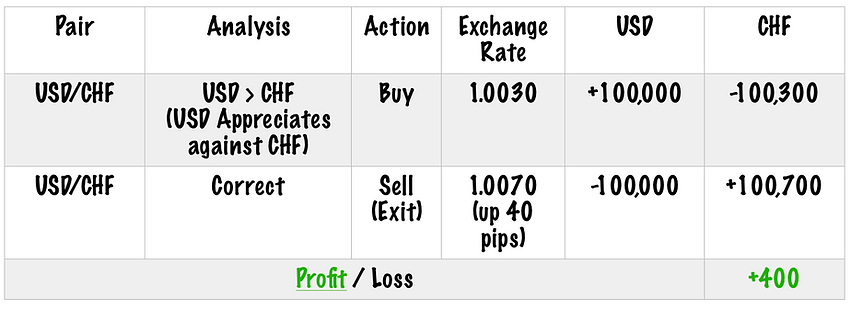top of page

# BUYING AND SELLING IN DETAILS

Base Currency

The first currency on the left is called "base currency"

Counter Curency

The second currency on the right is caled "counter Currency"

Ask price is the lowest price the broker will pay to sell the instrument.

Bid

Bid price is the highest price the broker will pay to purchase the instrument.

The difference between the Ask price and Bid price is broker’s profit that is called spread.

Pip

A pip is a standardised unit and is the smallest amount by which a currency quote can change. It is usually 0.0001 for U.S.-dollar related currency pairs, and 0.01 for Japanese yen related currency pairs

Pipette

A pipette equals 1/10 (one tenth) of a pip and it represents a fraction of 1/100,000 (one in hundred thousand).

Lot

Lot is the measurement for the units of currency you buy and sell. A standard lot represents 100,000 units of any currency, whereas a mini-lot represents 10,000 and a micro-lot represents 1,000 units of any currency.

Now that you have learned the basics and technical terms, we can discuss how buying and selling work in the Forex market by using the terms you just learned earlier.

To fully understand this topic, you must know what a pip is and how to calculate it, what is meant by a Lotleveragebid, ask and spread

As discussed before, the aim of forex trading is simple. Like any other form of speculation, you want to buy a currency at one price and sell it at a higher price (or sell a currency at one price and buy it at a lower price) to make a profit.

Currency appreciation is an increase in the value of one currency to another currency. On the other hand, currency depreciation is a decrease in the value of one currency in relation to another currency.

Generally speaking, If you think a currency is going to depreciate, then you sell it. But, conversely, If you think that a currency is going to appreciate, you buy it.

Let's take a look at this example:

Assume you have a USD-based trading account with a leverage of 100:1 that has 1000 US dollar capital in it.

Your analysis tells you that Euro is undervalued relative to the US dollar. You see an opportunity that Euro could appreciate against the US dollar in the short term, roughly around ten pips. Therefore, you decide to buy the Euro and wait till a ten-pip rise happens against the US dollar by risking only five pips.

But to calculate your profit and loss in order to manage your risk before you place your trade, you need to know a few things:

1) Pip value of EUR/USD for your USD based account:

\$0.10 for a micro lot (1,000)

\$1 for a mini lot (10,000)

\$10 for a standard lot (100,000)

Let's assume you decide to open a standard lot.

2) Commission charged by the broker

Let's assume your broker charges no commission.

Let's assume your account is a fixed spread account, and the spread on EUR/USD is fixed at one pip (0.0001).

Now that you know all the required information, assuming the current exchange rate is 1.1350, you can calculate your profit and loss if you decide to get in at this rate. It is important to do that in order to calculate your risk exposure and manage your trade size according to your risk tolerance. Thus, below is the pre-calculated potential net profit and loss of your trade:

• Rate after a 10 pip rise: 1.1350 + 0.0010

• Potential profit: (1.1360-1.1350)*100,000= 100 US dollar

• Cost: 0.0001*100,000= 10 US dollar

Net Profit: 100-20= 90 Dollar

• Rate after a 5 pip decrease: 1.1350 - 0.0005 = 1.1345

• Potential loss: (1.1350-1.1340)*100,000= 50 US dollar

• Cost: 0.0001*100,000= 10 US dollar

Net Loss= 50+10= 60 Dollar

Now let's take a look at the two trade examples on USD/CHF for a better understanding of profit and loss calculations.

1. Analysis: Let's assume that your analysis signals that the US Dollar will appreciate in value against the Swiss Franc.

2. Action: Therefore, you go ahead and buy 1 standard lot (100,000 units) of USD/CHF at the exchange rate of 1.0030 (ask rate), and you receive US\$ 100,000, which is worth CHF 100,300 at the exchange rate of 1.0030.

3. Result: Now, let's assume your prediction was right, and the USD appreciated against CHF, and the exchange rate became 1.0070, or to put it differently, the exchange rate rose by 40 pips.

4. Execution: Thus, due to the rise in the exchange rate, you decide to exit (sell) and close your position. Thus, you sell the US\$ 100,000 that you bought earlier at the new exchange rate (Bid rate) of 1.0070 and receive 100,700 of Swiss Franc (100,000 x 1.0070).

5. Conclusion: Ignoring the cost of trading such as spread and commissions, you profit CHF 400 (100,700-100,300).Now let’s analyze what would have happened if your prediction was wrong.

1. Analysis: Let’s assume that your analysis signals that the US Dollar will depreciate in value against the Swiss Franc.

2. Action: Therefore, you go ahead and sell 1 lot (100,000 units) of USD/CHF at the exchange rate of 1.0030 (Bid rate). You receive around CHF 100,300 (100,000 x 1.0030), which is worth USD\$ 100,000 at the given exchange rate of 1.0030.

3. Result: Now, let’s assume your prediction was wrong, and the USD instead appreciated against CHF, and the exchange rate became 1.0080, or to put it differently, the exchange rate rose by 50 pips.

4. Execution: Thus, you decide to cut the loss and close the sell position, and you exchange the CHF 100,300 at the new exchange rate of 1.0080 for US dollar and receive 99,503 (100,300/1.0080).

5. Conclusion: Ignoring the cost of trading, such as spread and commissions, you lose roughly CHF 500 (100,000-99,500).bottom of page